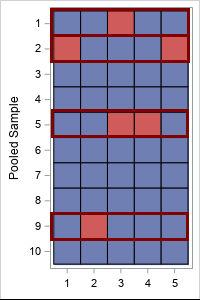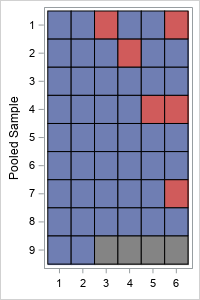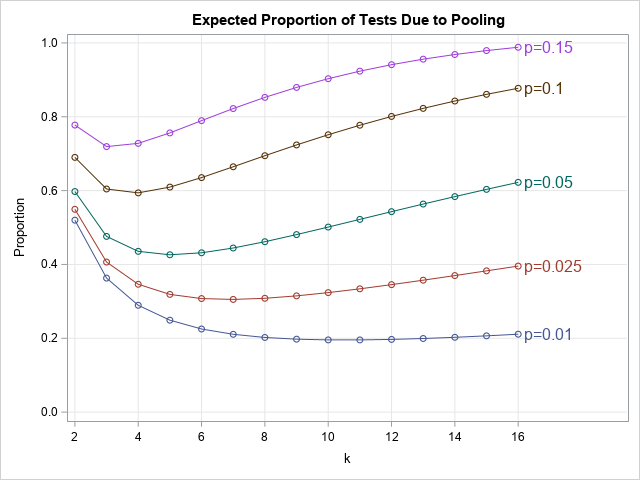Testing people for coronavirus is a public health measure that reduces the spread of coronavirus. Dr. Anthony Fauci, a US infectious disease expert, recently mentioned the concept of "pool testing." The verb "to pool" means "to combine from different sources." In a USA Today article, Dr. Deborah Birx, the coordinator for the White House coronavirus task force, suggests that pooling can increase the number of people who get tested tenfold. What is pool testing? How does it enable you to test more people while running fewer tests? This article looks at the mathematics and statistics of pool testing.

### What is pool testing?

Testing reduces the spread of coronavirus. As of 23Jun2020, the US tests about 500,000 people per day, according to an article in the MIT Technology Review. Tests are given sick or exposed people to see if they should quarantine themselves and alert others. Tests are given to health-care professionals and other front-line workers on a regular basis to ensure that these workers are healthy and can continue to serve the public. In short, it is important to be able to process massive numbers of tests every day. Pool testing can test more people with the same number of tests.

Pool testing is not a new idea. It has been used for more than a decade to ensure the safety of donated blood. When people donate blood, the blood bank tests the blood to make sure it does not contain viruses such as HIV, hepatitis, West Nile, or Zika. As the name implies, pool testing involves taking a portion of blood from multiple individuals and combining the portions into a pooled sample. (Reserve some blood from each individual in case you need a second test.) The viral test (usually nucleic acid testing, or NAT) is run on the pooled sample. If the test is negative, you conclude that all of the individual samples are negative. If the test is positive, you know that one or more of the individual samples is positive. You do not know which samples are positive, but that's okay because you reserved a portion of each sample. You can run a second round of tests on the reserved samples, one at a time, to discover which individual or individuals are infected.

There are several kinds of COVID-19 tests, but one type uses NAT on nasal secretions from a donor. I am a statistician, not a medical expert, but I assume that pooling nasal secretions are similar, in principle, to the idea of pooling blood samples. Of course, when you combine one positive sample with many negative samples, you dilute the amount of virus in the pooled sample. You do not want to get a false negative result, so the sensitivity of the test imposes a practical limitation on the number of samples that you can combine.

### An example of pool testingSuppose you have 50 samples that you want to test. If you test them individually, you need 50 tests. But suppose you combine five samples at a time into 10 pooled samples. In the first round of testing, you use 10 tests on the pooled samples. Suppose four of the tests are positive. In the second round of testing, you test the 4*5 = 20 samples that were in the four pooled samples. The total number of tests used is 30: 10 for the first round and 20 for the second round. You have tested the same number of samples while running 60% of the tests.

This is illustrated by the table at the right. The individual samples are the cells of the table. Blue is a negative sample; red is a positive sample. The rows represent the 10 pooled samples. The four highlighted rows (rows 1, 2, 5, and 9) indicate the pooled samples that test positive in the first round. The 20 samples in those rows must be tested individually in the second round.

Pool testing is most efficient when the probability of an infected sample is small. That enables you to pool many individuals at one time.

### How many tests are saved by using pool testing?

In the previous example, 50 individuals were tested by using 30 tests. That means that pool testing (with five samples in each pool) used only 60% of the tests as individual testing. The proportion of tests that you need is related to the probability of an infected sample (p) and how many tests (k) you combine to make a pooled sample.The lab that performs the tests cannot control the probability of an infected sample (p), but they can control the number of samples (k) in each pool. What happens if you change k? Let's revisit the previous example, but use six samples in each pool. The pooled-sample design for k=6 is shown to the right. There are nine pooled samples (rows) so nine tests are required for the first round. (Because 6 does not evenly divide 50, the last pooled sample only contains two samples.) Four pooled samples (rows 1, 2, 4, and 7) test positive, which triggers 4*k = 4*6 = 24 additional tests in the second round. So the total number of tests when k=6 is 9 + 24 = 33 tests.

Although pooling k=6 samples resulted in more total tests than for k=5 for these data, there is randomness in this result. The order of the samples and the number of positive samples are random and unknowable. Nevertheless, you can use probability theory to predict the expected number of tests that you will need in a random sample when you use k samples in each pool.

### How many samples should you combine to make a pooled sample?

If you know that the probability (p) that a sample is infected, you can compute the optimal number of samples (k) to combine into a pooled sample. Here "optimal" means "resulting in the fewest tests, on average."

Here comes the math. Suppose you want to test a large number, N, of individual samples. If each pooled sample contains k individual samples, then:

• There are about N/k pooled samples, so you need that many tests for the first round of testing.
• For each pooled sample, the probability that the sample does NOT test positive is the probability of having zero positive samples in a random set of k indpendent samples. This probability is given by the binomial distribution: Binom(0, p, k) = (1 – p)k. Consequently, the probability that a pooled sample DOES test positive is
p2 = 1 – (1 – p)k.
• From the preceding calculation, the expected number of positive pooled samples is p2N/k.
• Each positive test from the first round triggers k additional tests in the second round, so the expected number of tests in the second round is p2N.
• Consequently, the expected number of TOTAL tests is NTot = N(1/k + p2).
• If you don't use pooling, you have to do N tests, so pool testing reduces the total number of tests by the expected fraction 1/k + p2 or
f(k; p) = 1/k + 1 + (1 – p)k.

In summary, if p is the probability that a sample is infected, and you combine k samples into each pool, expected reduction in tests is the proportion 1/k + 1 + (1 – p)k.

The lab can control k, the number of samples that are combined into each pooled sample. So what value of k is expected to cost the fewest number of tests? The following graph shows a graph of the expected proportion as a function of k for several value of the proportion p.The graph shows the following:

• When p=0.15 (15% of tests are expected to be positive), the optimal value is k=3, and you can expect to need 72% of the tests compared to testing each individual sample.
• When p=0.1 (10% of tests are expected to be positive), the optimal value is k=4, and you need about 59% of the tests compared to not pooling.
• When p=0.05 (5%), the optimal value is k=5, and you need about 43% of the tests compared to not pooling.
• When p=0.025 (2.5%), the optimal value is k=7, and you need about 31% of the tests.
• When p=0.01 (1%), the optimal value is k=11, and you need about 20% of the tests.

In this section, I focused on testing the same number of people with fewer tests. But you can also test more people with the same number of tests. For example, when p=0.01, you can either test the same number of people using 20% of the tests, or you can test five times more people (because 1/0.2 = 5) with the same number of tests.

### Can pool testing reduce tests 'tenfold'?

In the USA Today article, epidemiologists are quoted as saying that "pool testing has the potential to increase the number of people tested tenfold, 'if not 100-fold.'” Notice that none of the curves in this article indicate an increase that large. The largest increase is for p=0.01 and k=11, which is a fivefold increase.

Pool testing is most effective when the probability of a positive test is very small. A small probability occurs when you test a mostly healthy population as a way of monitoring health and preventing future outbreaks. If the test is very sensitive, you could theoretically pool dozens or hundreds of samples together, which could lead to a dramatic increase in the number of people that you can test. I do not know the sensitivity of the COVID-19 tests in the US, but there is a practical limit on how many samples can be pooled.

Currently, many tests in the US are given to people who are exhibiting symptoms or believe they might have been exposed. For example, in North Carolina, the current percentage of positive tests is 6%. That is also the approximate national average. Some of the hardest-hit US states (Arizona, Florida, Texas,...) are reporting positive test rates closer to 10% or 15% or more, as reported by the Johns Hopkins data on "Daily State-by-State Testing Trends." Unfortunately, the hardest-hit states (where there is a high probability of a positive test) benefit the least from pooling.

In conclusion, this article shows that you can use pool testing to reduce the number of tests required to test a large number of people. If applied to the current testing in the US, it could reduce the number of tests 30% to 80%. (Equivalently, you could test 1.25 to 3.3 times as many people.) If applied to a mostly healthy population, it could reduce the number of tests even more. (Equivalently, test many more people.) This article is about the mathematics of pool testing and does not consider economic factors or practical considerations.

The post Pool testing: The math behind combining medical tests appeared first on The DO Loop.